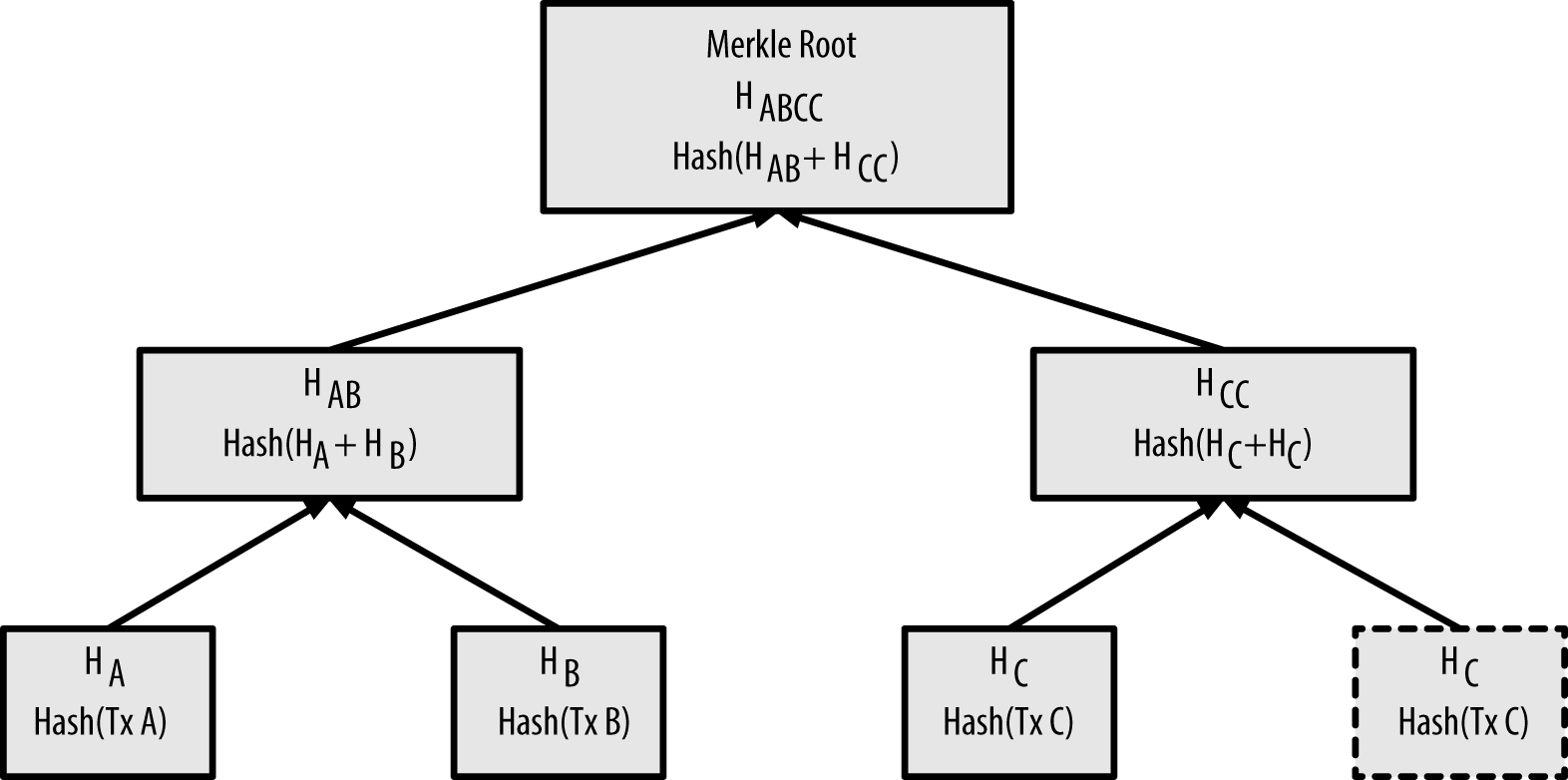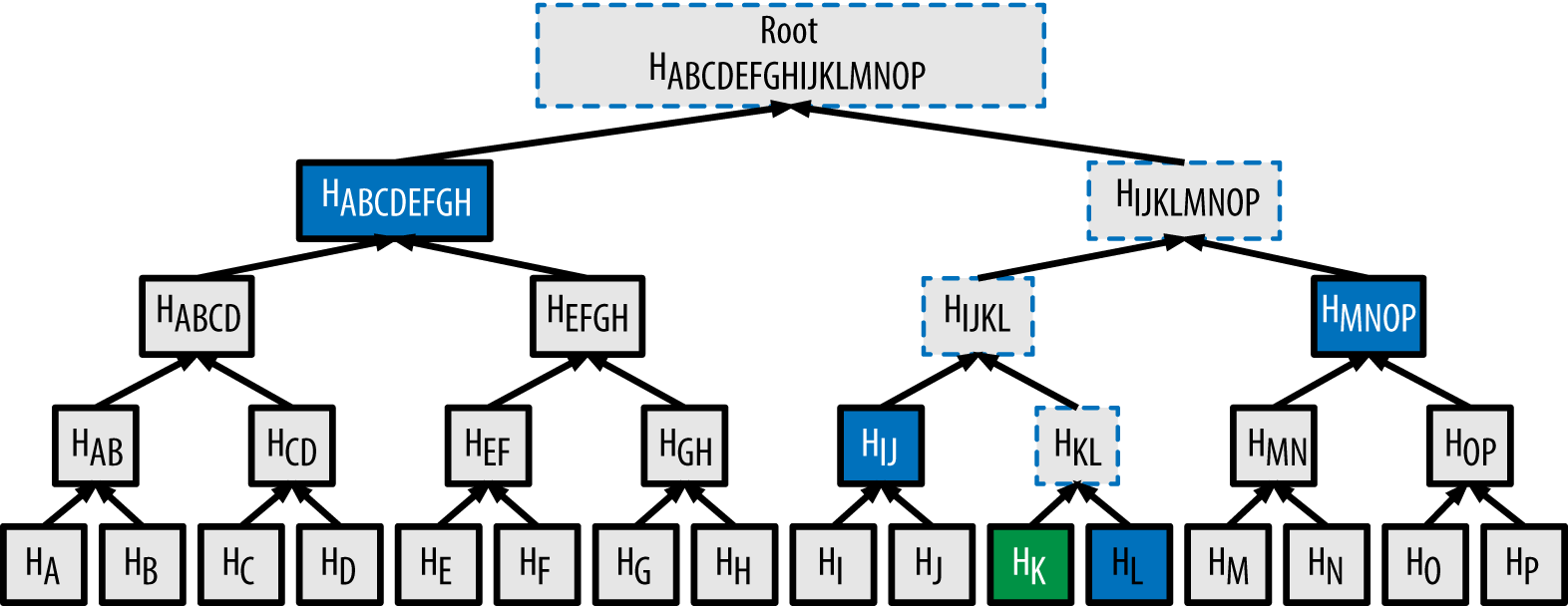# the block in blockchain explained (merkle trees)

This exposition focuses on a blockchain technique often used in a block: Merkle Trees.

setup:

``````{-# LANGUAGE NoImplicitPrelude #-}
{-# LANGUAGE OverloadedStrings #-}
{-# LANGUAGE ViewPatterns      #-}

module Block where

import           ClassyPrelude           as CP hiding ((<|))
import           Control.Monad.ST.Strict (runST)
import           Crypto.Hash.SHA256      as C  (hash)
import           Data.ByteString.Char8   as BS (pack, replicate)
import           Data.Char               (chr)
import           Data.Map.Strict         as M
import           Data.Sequence           as S
import           Data.STRef.Strict
import           Test.Hspec
import           Test.RandomStrings      (randomASCII, randomWord)

{-# ANN module ("HLint: ignore Eta reduce"::String)         #-}
{-# ANN module ("HLint: ignore Reduce duplication"::String) #-}``````

## block definition

For this exposition, a block will be defined as:

``````data Block = Block
{ header :: ! BlockHeader
, txs    :: ! Transactions
} deriving (Eq, Show)``````

where transactions are are an ordered sequence of data:

``type Transactions = Seq Transaction``

Here the “transactions” are uninterpreted opaque data:

``type Transaction  = ByteString``

In a “real” blockchain, this is where “smart contracts” would be involved (a subject of a future exposition).

The most important part, for now, is the header:

``````data BlockHeader = BlockHeader
{ bPrevHash   :: ! BHash      -- ^ hash of previous block
, bMerkleRoot :: ! BHash      -- ^ root hash of this block's transactions
, bTimestamp  :: ! BTimestamp -- ^ when this block was created
} deriving (Eq, Show)``````

where

``````type BHash      = ByteString
type BTimestamp = ByteString``````

The exposition The Chain in Blockchain explained how blocks are linked in chains using `bPrevHash` etc. The above definitions are to relate the current exposition to the previous exposition. They are not futher used below. Here the focus is on `bMerkleRoot`.

## merkle root

The merkle root is a hash of the hash of the bytes that make up each transaction.

The bytes of all transactions in the block are individually hashed, forming a list of hashes:

``````type HashDigest = ByteString
type Hashes     = Seq HashDigest

txHashes :: Block -> Hashes
txHashes (Block _ transactions) = CP.map C.hash transactions``````

Then each each adjacent pair of hashes are concatenated and hashed again,

``````concatHash :: HashDigest -> HashDigest -> HashDigest
concatHash x y = C.hash (x <> y)``````

forming the parent of those pairs. This process is repeated on each level of parents until there is a single node. That node is the “merkle root”:merkelroot

## creating a merkle root

The `createMerkleRoot` function below is written to mimic `create_merkle` in Mastering Bitcoin (scroll down to “Example 7-1. Building a merkle tree”). That code is a modification of the “real” `generate_merkel_root` code in libbitcoin

``````createMerkleRoot :: Hashes -> HashDigest
createMerkleRoot (viewl -> EmptyL) = nullHash
createMerkleRoot              hs0  = loop hs0
where
loop hs =
if S.length hs == 1 then
S.index hs 0
else
loop (combine (dupWhenOdd hs))

-- when odd, duplicate last hash
dupWhenOdd hs =
if odd \$ S.length hs then
hs |> S.index hs (S.length hs - 1)
else
hs

-- Make newHashes (1/2 size of given hashes)
-- where every element of newHashes is made
-- by taking adjacent pairs of given hashes,
-- concatenating their contents
-- then hashing that concatenated contents.
combine hs = runST \$ do
newHashes <- newSTRef S.empty
forM_ (S.chunksOf 2 hs) \$ \x ->
modifySTRef' newHashes (|> concatHash (S.index x 0) (S.index x 1))
readSTRef newHashes

nullHash :: HashDigest
nullHash = BS.replicate 32 (chr 0)

t1 :: Spec
t1 =
let one   = S.empty |> C.hash "01"
two   = one     |> C.hash "10"
three = two     |> C.hash "11"
in describe "t1" \$ do
it "empty" \$ createMerkleRoot S.empty `shouldBe` nullHash
it "one"   \$ createMerkleRoot one     `shouldBe` C.hash "01"
it "two"   \$ createMerkleRoot two     `shouldBe` concatHash (C.hash "01") (C.hash "10")
it "three" \$ createMerkleRoot three   `shouldBe`
"\STX\SYN\n\251\228\227\135\246\SUB\EM\128\196\r\b\166\RS\NAK9\235X\236R\184\134\220\141wP\225\ACK\169\254"``````

The merkle root, besides acting as a “signature” of the transactions, can be used by lightweight peers to ensure that a transaction is in a block without needing to access (i.e., over a network connection) all the transactions in a block (e.g., Bitcoin’s Simplified Payment Verification).

## merkle path

A lightweight peer contacts a full peer asking for a “merkle path” from a particular transaction, through the merkle tree, to the merkle root.merkletreeimage

Assume the lightweight peer has transaction `K`. To verify that `K` is a member of the block it hashes transaction `K`, forming `H_K`. It sends `H_K` to a full peer. The full peer creates a merkle path by identifying which ordered set of hashes (marked in blue) it needs to send to the lightweight peer. The lightweight peer then uses its `K` along with the returned merkle path to hash through that path to reach the root. If resulting hash matches the root then the transaction is verified to be in the block, otherwise not.

The ordered hashes needed by the lightweight peer to hash from its transaction to the root are:

``H_L, H_IJ, H_MNOP, H_ABCDEFGH``

## creating a merkle path

To create a merkle path an explicit tree may be used:

``````-- | Left or Right neighbor
type MerklePathElement = Either HashDigest HashDigest

-- | neighbor and parent are `Nothing` for the root
data MerkleInfo = MerkleInfo
{ identity :: ! HashDigest                -- ^ for testing
, neighbor :: ! (Maybe MerklePathElement)
, parent   :: ! (Maybe HashDigest)
} deriving (Eq, Show)

type MerkleTreeMap = M.Map HashDigest MerkleInfo

-- | This function has the same structure as `createMerkleRoot`.
-- The difference is that this one creates a tree (using a Map).
createMerkleTreeMap :: Hashes -> MerkleTreeMap
createMerkleTreeMap (viewl -> EmptyL) = M.empty
createMerkleTreeMap              hs0  = loop (hs0, M.empty)
where
loop (hs, m) =
if S.length hs == 1 then
let h = S.index hs 0
in M.insert h (MerkleInfo h Nothing Nothing) m
else
loop (combine (dupWhenOdd hs) m)

dupWhenOdd hs =
if odd \$ S.length hs then
hs |> S.index hs (S.length hs - 1)
else
hs

combine hs m = runST \$ do
newHashesAndMap <- newSTRef (S.empty, m)
forM_ (S.chunksOf 2 hs) \$ \x -> do
let parentHash = concatHash (S.index x 0) (S.index x 1)
leftHash   = S.index x 0
rightHash  = S.index x 1
l          = MerkleInfo leftHash  (Just (Right rightHash)) (Just parentHash)
r          = MerkleInfo rightHash (Just (Left  leftHash))  (Just parentHash)
modifySTRef' newHashesAndMap (\(hs', m') ->
( hs' |> parentHash
, M.insert leftHash l (M.insert rightHash r m')))
readSTRef newHashesAndMap``````

Then, given a hash of a transaction and the tree created above, create a path to the root.

``````type MerklePath = Seq MerklePathElement

merklePathTo :: HashDigest -> MerkleTreeMap -> MerklePath
merklePathTo h m = go (m ! h) S.empty
where
go (MerkleInfo _              _    Nothing) xs = CP.reverse xs
go (MerkleInfo _ (Just (Left  l)) (Just p)) xs = go (m ! p) (Left  l <| xs)
go (MerkleInfo _ (Just (Right r)) (Just p)) xs = go (m ! p) (Right r <| xs)
go  MerkleInfo {}                            _ = error "merklePathTo"

t2 :: Spec
t2 = do
-- create TXs A .. O
txs' <- runIO (S.replicateM 15 (do rw <- randomWord randomASCII 100; return (BS.pack rw)))
-- create TX hashes H_A .. H_O (see the diagram above)
let hashes = CP.map C.hash txs'
hkData = S.index txs' 10
hK'    = C.hash hkData
-- this will dup H_O to add H_P
m      = createMerkleTreeMap hashes
-- manually create the pieces needed for the merkle path for H_K
(MerkleInfo _ (Just (Right       hL)) (Just hKL))               = m ! hK'
(MerkleInfo _ (Just (Left       hIJ)) (Just hIJKL))             = m ! hKL
(MerkleInfo _ (Just (Right    hMNOP)) (Just hIJKLMNOP))         = m ! hIJKL
(MerkleInfo _ (Just (Left hABCDEFGH)) (Just hABCDEFGHIJKLMNOP)) = m ! hIJKLMNOP
describe "t2" \$ do
it "root" \$ createMerkleRoot hashes `shouldBe` hABCDEFGHIJKLMNOP
it "merklePath" \$
merklePathTo hK' (createMerkleTreeMap hashes) `shouldBe`
S.empty |> Right hL |> Left hIJ |> Right hMNOP |> Left hABCDEFGH
it "createMerkleTreeMap" \$
createMerkleTreeMap (S.empty |> "00" |> "01" |> "02" |> "03")
`shouldBe`
M.fromList [("00",MerkleInfo { identity = "00", neighbor = Just (Right "01")
, parent = Just "\136\139\EM\164;\NAK\SYN\131\200x\149\246!\GS\159\134@\249{\220\142\243/\ETX\219\224W\200\245\229m2"})
,("01",MerkleInfo { identity = "01", neighbor = Just (Left "00")
, parent = Just "\136\139\EM\164;\NAK\SYN\131\200x\149\246!\GS\159\134@\249{\220\142\243/\ETX\219\224W\200\245\229m2"})
,("02",MerkleInfo { identity = "02", neighbor = Just (Right "03")
, parent = Just "\194Wm\216T\SUB\"\\\206\SOHTu\226\213\171\186\201\159\${\145DzS\137\130n+\198'@\192"})
,("03",MerkleInfo { identity = "03", neighbor = Just (Left "02")
, parent = Just "\194Wm\216T\SUB\"\\\206\SOHTu\226\213\171\186\201\159\${\145DzS\137\130n+\198'@\192"})
,("\136\139\EM\164;\NAK\SYN\131\200x\149\246!\GS\159\134@\249{\220\142\243/\ETX\219\224W\200\245\229m2",MerkleInfo {identity = "\136\139\EM\164;\NAK\SYN\131\200x\149\246!\GS\159\134@\249{\220\142\243/\ETX\219\224W\200\245\229m2", neighbor = Just (Right "\194Wm\216T\SUB\"\\\206\SOHTu\226\213\171\186\201\159\${\145DzS\137\130n+\198'@\192"), parent = Just "\156\160c\144\$\227\138Z\254|x\231wk\DC3 \228;\235\130:\200\DLE\\0 \131\134w\130\163\243"})
,("\194Wm\216T\SUB\"\\\206\SOHTu\226\213\171\186\201\159\${\145DzS\137\130n+\198'@\192",MerkleInfo {identity = "\194Wm\216T\SUB\"\\\206\SOHTu\226\213\171\186\201\159\${\145DzS\137\130n+\198'@\192", neighbor = Just (Left "\136\139\EM\164;\NAK\SYN\131\200x\149\246!\GS\159\134@\249{\220\142\243/\ETX\219\224W\200\245\229m2"), parent = Just "\156\160c\144\$\227\138Z\254|x\231wk\DC3 \228;\235\130:\200\DLE\\0 \131\134w\130\163\243"})
,("\156\160c\144\$\227\138Z\254|x\231wk\DC3 \228;\235\130:\200\DLE\\0 \131\134w\130\163\243",MerkleInfo {identity = "\156\160c\144\$\227\138Z\254|x\231wk\DC3 \228;\235\130:\200\DLE\\0 \131\134w\130\163\243", neighbor = Nothing, parent = Nothing})]``````

## using a merkle path

Once the light peer receives the merkle path from the full peer it can verify that a transaction is in the block:

``````isTxInBlock :: Transaction -> MerklePath -> HashDigest -> Bool
isTxInBlock tx mp merkleRoot = loop (C.hash tx) mp == merkleRoot
where
loop h (viewl -> S.EmptyL)        = h
loop h (viewl -> (Left  x :< xs)) = go x h xs
loop h (viewl -> (Right x :< xs)) = go h x xs
loop _                         _  = error "isTxInBlock"
go x y xs                         = loop (concatHash x y) xs

t3 :: Spec
t3 = do
txs' <- runIO (S.replicateM 15 (do rw <- randomWord randomASCII 100; return (BS.pack rw)))
let hashes     = CP.map C.hash txs'
notHKTX    = S.index txs'  9
hKTX       = S.index txs' 10
hK         = C.hash hKTX
merkleRoot = createMerkleRoot hashes
merklePath = merklePathTo hK (createMerkleTreeMap hashes)
describe "t3" \$ do
it "hK" \$
C.hash (S.index txs' 10) `shouldBe` hK
it "isTxInBlock True"  \$
isTxInBlock    hKTX merklePath merkleRoot `shouldBe` True
it "isTxInBlock False" \$
isTxInBlock notHKTX merklePath merkleRoot `shouldBe` False``````

## summary

• a block may contain a merkle root : essentially a signature of its data (e.g., “transactions”)
• the hash makes it possible to detect changes to the data
• the merkle root also enables lightweight peers to verify a particular transaction is in a block without needing access to all transactions in a block

## credits

Diagrams from Mastering Bitcoin by Andreas M. Antonopoulos.

Thanks to Ulises Cerviño Beresi, Victor Cacciari Miraldo, Alejandro Serra Mena and Mark Moir for pre-publication feedback.

## source code and discussion

The code for this exposition is available at github

run the code: `stack test`

A discussion is at: reddit

comments powered by Disqus